# The arithmetic mean

The arithmetic mean of numbers is the sum of these numbers divided by the number of terms.

 arithmetic mean = sum of all numbers / number of terms

To find the arithmetic mean of numbers, add these numbers and divide the result by the number of numbers. ( In the case of finding the arithmetic mean of two numbers, divide by 2, in the case of three 3, etc.)

### Examples of finding the arithmetic mean of two numbers

Example 1: Find the arithmetic mean of two numbers: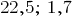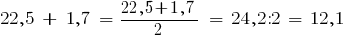Example 2: Find the arithmetic mean of the three numbers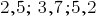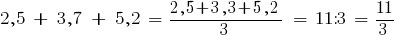Example 3: Find the average of four numbers: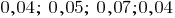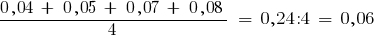### The problem of finding the arithmetic mean

Task 1: while travelling in the car for the first hour passed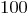, away, for the second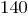km and for third and fourth —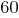what is the average per hour drove the car?

In this task, on the average it is necessary to find average of numbers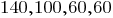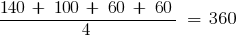— average miles per hour drove the car.

Task 2: Candy red poppy stand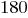UAH per kg, and the starry night -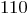UAH per kg. Marina bought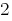kg of a red poppy, a pound starry night. And Alenka bought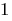kg of a red poppy and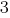kg starry night. What is the average purchase price of Marinka and Alenka?

Marina spent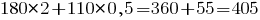Helen spent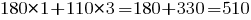In this task, on the average it is necessary to find average of numbers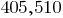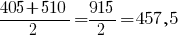— an average of UAH spent Marinka and Alenka.

Tags:
Chapter:
Versions in other languages:
Share with friends: